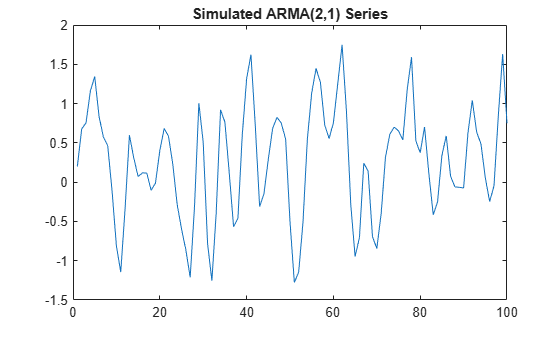# Choose ARMA Lags Using BIC

This example shows how to use the Bayesian information criterion (BIC) to select the degrees p and q of an ARMA model. Estimate several models with different p and q values. For each estimated model, output the loglikelihood objective function value. Input the loglikelihood value to `aicbic` to calculate the BIC measure of fit (which penalizes for complexity).

### Simulate ARMA time series

Simulate an ARMA(2,1) time series with 100 observations.

```Mdl0 = arima('Constant',0.2,'AR',{0.75,-0.4},... 'MA',0.7,'Variance',0.1); rng(5) Y = simulate(Mdl0,100); figure plot(Y) xlim([0,100]) title('Simulated ARMA(2,1) Series')```### Plot Sample ACF and PACF

Plot the sample autocorrelation function (ACF) and partial autocorrelation function (PACF) for the simulated data.

```figure subplot(2,1,1) autocorr(Y) subplot(2,1,2) parcorr(Y)```Both the sample ACF and PACF decay relatively slowly. This is consistent with an ARMA model. The ARMA lags cannot be selected solely by looking at the ACF and PACF, but it seems no more than four AR or MA terms are needed.

### Fit ARMA(p,q) Models to Data

To identify the best lags, fit several models with different lag choices. Here, fit all combinations of p = 1,...,4 and q = 1,...,4 (a total of 16 models). Store the loglikelihood objective function and number of coefficients for each fitted model.

```LogL = zeros(4,4); % Initialize PQ = zeros(4,4); for p = 1:4 for q = 1:4 Mdl = arima(p,0,q); [EstMdl,~,LogL(p,q)] = estimate(Mdl,Y,'Display','off'); PQ(p,q) = p + q; end end```

### Calculate BIC

Calculate the BIC for each fitted model. The number of parameters in a model is p + q + 1 (for the AR and MA coefficients, and constant term). The number of observations in the data set is 100.

```logL = LogL(:); pq = PQ(:); [~,bic] = aicbic(logL,pq+1,100); BIC = reshape(bic,4,4)```
```BIC = 4×4 102.4215 96.2339 100.8005 100.3440 89.1130 93.4895 97.1530 94.0615 93.6770 93.2838 100.2190 103.4779 98.2820 102.0442 100.3024 107.5245 ```

In the output BIC matrix, the rows correspond to the AR degree (p) and the columns correspond to the MA degree (q). The smallest value is best.

`minBIC = min(BIC,[],'all')`
```minBIC = 89.1130 ```
`[minP,minQ] = find(minBIC == BIC)`
```minP = 2 ```
```minQ = 1 ```

The smallest BIC value is `89.1130` in the (2,1) position. This corresponds to an ARMA(2,1) model, which matches the model that generated the data.